Grid Mapping Fractals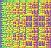# Fractals

The Mandelbrot Set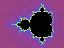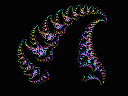Flower
Big bugs have little bugs
On their backs to bite 'em,
And these bugs have smaller bugs,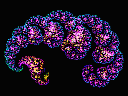Plume

A fractal is a geometric object which has two important characteristics:

• It is recursive; that is, the process of its creation gets repeated indefinitely; and
• It is self-similar; that is, copies of the entire fractal may be found, in reduced form, within the fractal.

These images were created by a group of Visual Basic programs. All of them use the "double triangle" algorithm.

#### "Double Triangle" Fractals

The "double triangle" algorithm creates fractals in the following manner: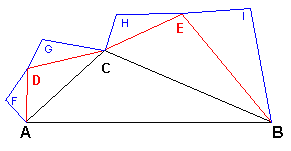Begin with line segmentAB, and add point C to form a triangle. Repeat the same process by forming similar triangles on line segments AC and CB, creating points D, and E. Once again, repeat the process of creating similar triangles on the new line segments AD, DC, CE, and EB, giving rise to points F, G, H, and I.

Continue in this manner until such time as a line segment becomes shorter than a pre-defined length (usually less than one pixel in the display), and then plot a point at the midpoint of that line. Colors may vary according to how many times the triangles have been divided, that is, the "depth" of division at that point.

A true fractal would never stop dividing, of course, but would continue to infinity.

Fractals

Last Updated November 18th, 2001
Web Page by Ned May
URL http://chromatism.net/chromat/fractals.htm